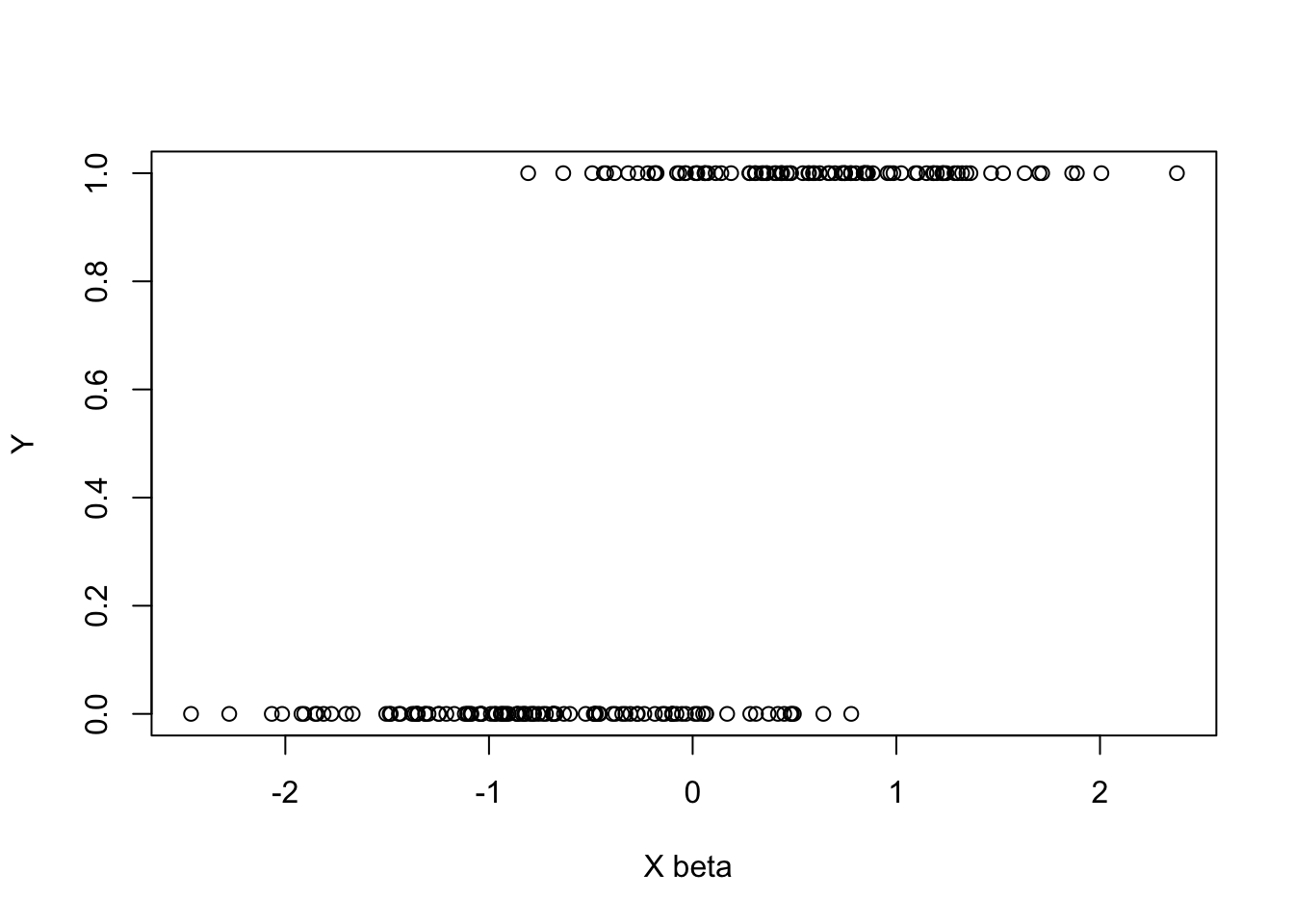In this article, we discuss the R code that computes the maximum score estimator proposed in C. Manski (1975) and C. F. Manski (1985). In the follwoing we consider the simulation scenario used in Table 2 of Patra, Seijo, and Sen (2015). We fix $$\beta_0=\frac{1}{\sqrt{d}}(1,\ldots,1)^\top$$ and assume that $U|X\sim N\left(0,\frac{1}{(1+|X|^2)^{2}}\right) , \quad X\sim \mbox{Uniform}([-1,1]^d), \quad \mbox{ and} \quad Y = \mathbf{1}_{\beta_0^\top X + U\geq 0}.$ For demonstration purposes, we fix $$n=200$$ and $$d=3$$. The following codes generate the sample and computes the maximum score estimator.

rm(list= ls())
source('MSE.R')
## Loading required package: slam
n<-200
d<-3
X <- matrix(runif(n*d, -1,1), n,d)
beta.0 <- rep(1,d)
sdx <- (1 +  rowSums(X^2))^(-1)
err <- rnorm(n,0,sdx)
ind <- X%*%beta.0
y <- as.vector((ind+err>0)*1)
plot(ind,y, xlab= paste(" X", expression(beta)), ylab="Y")ans <- MSE(X,y)
cat(" The MSE for this data set is:  ")
print(ans$beta.hat, digits=3) print(paste("It took ", format(ans$time,  digits=2), " secs to compute the above MSE"))
## Rcplex: num variables=203 num constraints=200
##  The MSE for this data set is:   0.642 0.677 0.360
##  "It took  0.27 secs  secs to compute the above MSE"

The above code requires a valid CPLEX installation and the R pakcage Rcplex to run. Our codes are based on the excact MIP algorithm propsoed in Florios and Skouras (2008). The required R file MSE.R’’ can be downloaded here. The codes to implement the smoothed botstarp procedure in Patra, Seijo, and Sen (2015) is forthcoming.

I would like to thank Kostas Florios for his helpful discussions and comments. See here for a MATLAB version of the code.